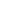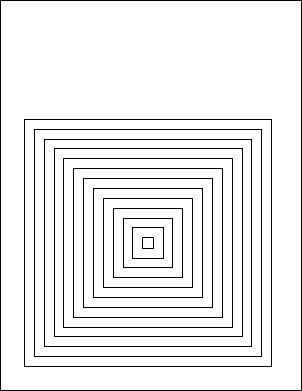Type Default Read Only Description [C#] `XRect` [Visual Basic] `XRect` The dimensions of the current page. No The current rectangle used for drawing operations.Notes

 This property determines the current rectangle. This is used by a number of operations including AddText, AddImage, FrameRect and FillRect. The XRect object represents a rectangular area in two-dimensional space. The properties of the XRect object represent the bottom left and top right corners of the area. AddText adds text within the current rectangle wrapping the text at the edges. The AddImage methods add an image scaled to fill the current rectangle. FrameRect frames the current rectangle and FillRect fills the current rectangle. When you change this property the Pos property is reset to point to the top left of the Rect.Example

 The following code creates a PDF document containing a number of concentric frames.   ```using (Doc doc = new Doc()) {   doc.Rect.String = "50 50 550 550";   for (int i = 1; i <= 20; i++) {     doc.FrameRect();     doc.Rect.Inset(20, 20);   }   doc.Save(Server.MapPath("docrect.pdf")); } ``` ```Using doc As New Doc()   doc.Rect.String = "50 50 550 550"   Dim i As Integer = 1   While i <= 20     doc.FrameRect()     doc.Rect.Inset(20, 20)     System.Math.Max(System.Threading.Interlocked.Increment(i),i - 1)   End While   doc.Save(Server.MapPath("docrect.pdf")) End Using ```docrect.pdf